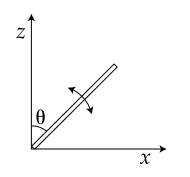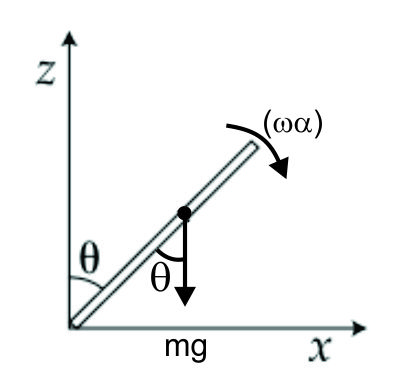#### A slender uniform rod of mass M and lengthis pivoted at one end so that it can rotate in a vertical plane (see figure).  There is negligible friction at the pivot.  The free end is held vertically above the pivot and then released.  The angular acceleration of the rod when it makes an angle θ with the vertical is :Option 1)Option 2)Option 3)Option 4)As we learnt in

Analogue of second law of motion for pure rotation -- wherein

Torque equation can be applied only about two point

(i) centre of motion.

(ii) point which has zero velocity/acceleration.Torque at an angleOption 1)This is the correct option.

Option 2)This is an incorrect option.

Option 3)This is an incorrect option.

Option 4)This is an incorrect option.

#### perimeter